Next: Results Up: A dynamical model for Previous: Heart rate variability

# The dynamical model

The model generates a trajectory in a three-dimensional state space with co-ordinates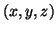. Quasi-periodicity of the ECG is reflected by the movement of the trajectory around an attracting limit cycle of unit radius in the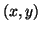-plane. Each revolution on this circle corresponds to one RR-interval or heart beat. Inter-beat variation in the ECG is reproduced using the motion of the trajectory in the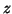-direction. Distinct points on the ECG, such as the P,Q,R,S and T are described by events corresponding to negative and positive attractors/repellors in the-direction. These events are placed at fixed angles along the unit circle given by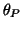,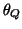,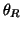,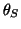and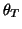(see Fig. 2). When the trajectory approaches one of these events, it is pushed upwards or downwards away from the limit cycle, and then as it moves away it is pulled back towards the limit cycle. The dynamical equations of motion are given by a set of three ordinary differential equations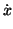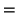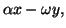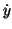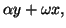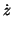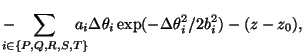(1)

where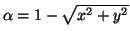,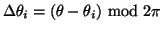,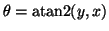and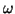is the angular velocity of the trajectory as it moves around the limit cycle. Baseline wander was introduced by coupling the baseline value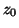in (1) to the respiratory frequency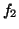using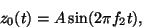(2)

where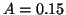mV. These equations of motion given by (1) were integrated numerically using a fourth order Runge-Kutta method  with a fixed time step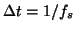where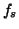is the sampling frequency. Visual analysis of a section of typical ECG from a normal subject was used to suggest suitable times (and therefore angles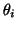) and values of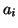and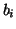for the PQRST points. The times and angles are specified relative to the position of the R-peak as shown in Table I. A trajectory generated by equation (1) in three-dimensions corresponding tois illustrated in Fig. 2. This demonstrates how the positions of the events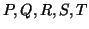act on the trajectory in the-direction as it precesses around the unit circle in the-plane. Thevariable from the three-dimensional system (1) yields a synthetic ECG with realistic PQRST morphology (Fig. 3). The similarity between the synthetic ECG and the real ECG may be seen by comparing Fig. 3 with Fig. 1. Note that noise has not been added to the model at this point.

 Index (i) P Q R S T Time (secs) -0.2 -0.05 0 0.05 0.3(radians)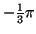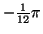0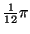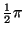1.2 -5.0 30.0 -7.5 0.750.25 0.1 0.1 0.1 0.4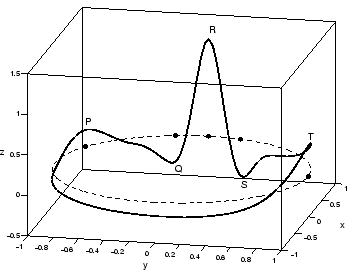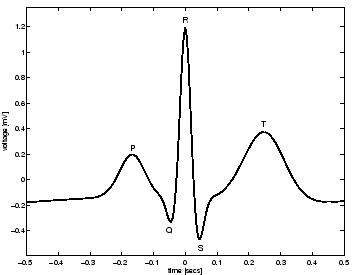By contrasting the dynamical model (1) with the mechanisms underlying the cardiac cycle, it is obvious that the time required to complete one lap of the limit cycle is equal to the RR-interval of the synthetic ECG signal. Variations in the length of the RR-intervals can be incorporated by varying the angular velocity. The effects of both RSA and Mayer waves in the power spectrum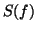of the RR-intervals are incorporated by generating RR-intervals which have a bimodal power spectrum consisting of the sum of two Gaussian distributions,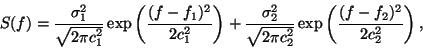(3)

with means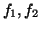and standard deviations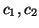. Power in the LF and HF bands are given by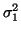and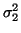respectively whereas the variance equals the total area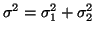, yielding an LF/HF ratio of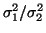. Fig. 4 shows the power spectrumgiven by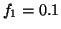,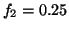,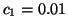,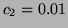and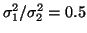. The Gaussian frequency distribution is motivated by the typical power spectrum of a real RR tachogram .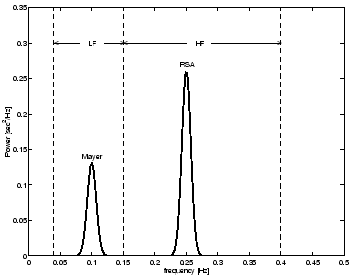A RR-interval time series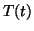with power spectrumis generated by taking the inverse Fourier transform of a sequence of complex numbers with amplitudes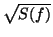and phases which are randomly distributed between 0 and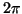. By multiplying this time series by an appropriate scaling constant and adding an offset value, the resulting time series can be given any required mean and standard deviation. Suppose thatrepresents the time series generated by the RR-process with power spectrum. The time-dependent angular velocity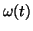of motion around the limit cycle is then given by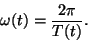(4)

In this way the series of RR-intervals of the resultant synthetic ECG will also have a power spectrum equal to; this will be demonstrated in the next section.Next: Results Up: A dynamical model for Previous: Heart rate variability
2003-10-08